# Square

For other uses, see Square (disambiguation).
Square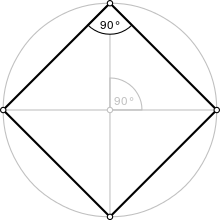Type Regular polygon
Edges and vertices 4
Schläfli symbol {4}
Coxeter diagramSymmetry group Dihedral (D4), order 2×4
Internal angle (degrees) 90°
Dual polygon Self
Properties Convex, cyclic, equilateral, isogonal, isotoxal

In geometry, a square is a regular quadrilateral, which means that it has four equal sides and four equal angles (90-degree angles, or right angles). It can also be defined as a rectangle in which two adjacent sides have equal length. A square with vertices ABCD would be denotedABCD.

## Properties

A square is a special case of a rhombus (equal sides, opposite equal angles), a kite (two pairs of adjacent equal sides), a parallelogram (opposite sides parallel), a quadrilateral or tetragon (four-sided polygon), and a rectangle (opposite sides equal, right-angles) and therefore has all the properties of all these shapes, namely:

• The diagonals of a square bisect each other and meet at 90°
• The diagonals of a square bisect its angles.
• Opposite sides of a square are both parallel and equal in length.
• All four angles of a square are equal. (Each is 360°/4 = 90°, so every angle of a square is a right angle.)
• All four sides of a square are equal.
• The diagonals of a square are equal.
• The square is the n=2 case of the families of n-hypercubes and n-orthoplexes.
• A square has Schläfli symbol {4}. A truncated square, t{4}, is an octagon, {8}. An alternated square, h{4}, is a digon, {2}.

### Perimeter and area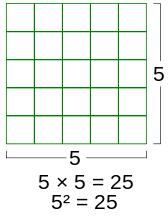The area of a square is the product of the length of its sides.

The perimeter of a square whose four sides have lengthisand the area A isIn classical times, the second power was described in terms of the area of a square, as in the above formula. This led to the use of the term square to mean raising to the second power.

The area can also be calculated using the diagonal d according toIn terms of the circumradius R, the area of a square issince the area of the circle isthe square fills approximately 0.6366 of its circumscribed circle.

In terms of the inradius r, the area of the square isBecause it is a regular polygon, a square is the quadrilateral of least perimeter enclosing a given area. Dually, a square is the quadrilateral containing the largest area within a given perimeter. Indeed, if A and P are the area and perimeter enclosed by a quadrilateral, then the following isoperimetric inequality holds:with equality if and only if the quadrilateral is a square.

A convex quadrilateral with successive sides a, b, c, d is a square if and only if :Corollary 15### Other facts

• The diagonals of a square are(about 1.414) times the length of a side of the square. This value, known as the square root of 2 or Pythagoras' constant, was the first number proven to be irrational.
• A square can also be defined as a parallelogram with equal diagonals that bisect the angles.
• If a figure is both a rectangle (right angles) and a rhombus (equal edge lengths), then it is a square.
• If a circle is circumscribed around a square, the area of the circle is(about 1.5708) times the area of the square.
• If a circle is inscribed in the square, the area of the circle is(about 0.7854) times the area of the square.
• A square has a larger area than any other quadrilateral with the same perimeter.
• A square tiling is one of three regular tilings of the plane (the others are the equilateral triangle and the regular hexagon).
• The square is in two families of polytopes in two dimensions: hypercube and the cross polytope. The Schläfli symbol for the square is {4}.
• The square is a highly symmetric object. There are four lines of reflectional symmetry and it has rotational symmetry of order 4 (through 90°, 180° and 270°). Its symmetry group is the dihedral group D4.
• If the inscribed circle of a square ABCD has tangency points E on AB, F on BC, G on CD, and H on DA, then for any point P on the inscribed circle,• Ifis the distance from an arbitrary point in the plane to the i-th vertex of a square andis the circumradius of the square, then## Coordinates and equations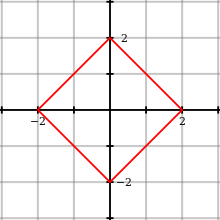plotted on Cartesian coordinates.

The coordinates for the vertices of a square with vertical and horizontal sides, centered at the origin and with side length 2 are (±1, ±1), while the interior of this square consists of all points (xi, yi) with −1 < xi < 1 and −1 < yi < 1. The equationspecifies the boundary of this square. This equation means "x2 or y2, whichever is larger, equals 1." The circumradius of this square (the radius of a circle drawn through the square's vertices) is half the square's diagonal, and equals. Then the circumcircle has the equationAlternatively the equationcan also be used to describe the boundary of a square with center coordinates (a, b) and a horizontal or vertical radius of r.

## Construction

The following animations show how to construct a square using a compass and straightedge. This is possible as 4 = 22, a power of two.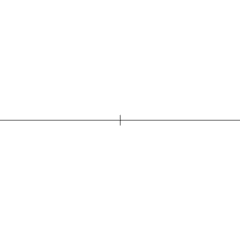Square at a given circumcircle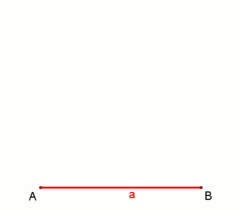Square at a given side length,
right angle by using Thales' theorem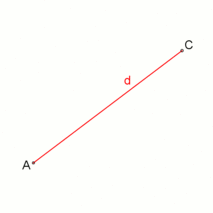Square at a given diagonal

## Symmetry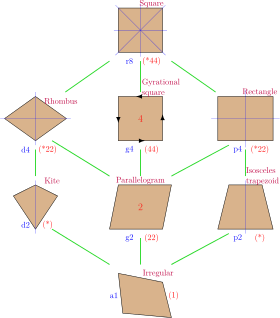The dihedral symmetries are divided depending on whether they pass through vertices (d for diagonal) or edges (p for perpendiculars) Cyclic symmetries in the middle column are labeled as g for their central gyration orders. Full symmetry of the square is r12 and no symmetry is labeled a1.

The square has Dih4 symmetry, order 8. There are 2 dihedral subgroups: Dih2, Dih1, and 3 cyclic subgroups: Z4, Z2, and Z1.

A square is a special case of many lower symmetry convex quadrilaterals:

• a rectangle with two adjacent equal sides
• a quadrilateral with four equal sides and four right angles
• a parallelogram with one right angle and two adjacent equal sides
• a rhombus with a right angle
• a rhombus with all angles equal
• a quadrilateral where the diagonals are equal and are the perpendicular bisectors of each other, i.e. a rhombus with equal diagonals

These 6 symmetries express 8 distinct symmetries on a square. John Conway labels these by a letter and group order.

Each subgroup symmetry allows one or more degrees of freedom for irregular quadrilaterals. r8 is full symmetry of the square, and a1 is no symmetry. d4, is the symmetry of a rectangle and p4, is the symmetry of a rhombus. These two forms are duals of each other and have half the symmetry order of the square. d2 is the symmetry of an isosceles trapezoid, and p2 is the symmetry of a kite. g2 defines the geometry of a parallelogram.

Only the g4 subgroup has no degrees of freedom but can seen as a square with directed edges.

## Squares inscribed in triangles

Main article: Triangle § Squares

Every acute triangle has three inscribed squares (squares in its interior such that all four of a square's vertices lie on a side of the triangle, so two of them lie on the same side and hence one side of the square coincides with part of a side of the triangle). In a right triangle two of the squares coincide and have a vertex at the triangle's right angle, so a right triangle has only two distinct inscribed squares. An obtuse triangle has only one inscribed square, with a side coinciding with part of the triangle's longest side.

The fraction of the triangle's area that is filled by the square is no more than 1/2.

## Squaring the circle

Squaring the circle is the problem, proposed by ancient geometers, of constructing a square with the same area as a given circle by using only a finite number of steps with compass and straightedge.

In 1882, the task was proven to be impossible, as a consequence of the Lindemann–Weierstrass theorem which proves that pi (π) is a transcendental number, rather than an algebraic irrational number; that is, it is not the root of any polynomial with rational coefficients.

## Non-Euclidean geometry

In non-Euclidean geometry, squares are more generally polygons with 4 equal sides and equal angles.

In spherical geometry, a square is a polygon whose edges are great circle arcs of equal distance, which meet at equal angles. Unlike the square of plane geometry, the angles of such a square are larger than a right angle. Larger spherical squares have larger angles.

In hyperbolic geometry, squares with right angles do not exist. Rather, squares in hyperbolic geometry have angles of less than right angles. Larger hyperbolic squares have smaller angles.

Examples: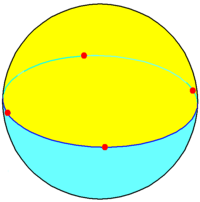Two squares can tile the sphere with 2 squares around each vertex and 180-degree internal angles. Each square covers an entire hemisphere and their vertices lie along a great circle. This is called a spherical square dihedron. The Schläfli symbol is {4,2}.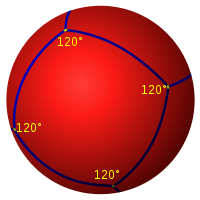Six squares can tile the sphere with 3 squares around each vertex and 120-degree internal angles. This is called a spherical cube. The Schläfli symbol is {4,3}.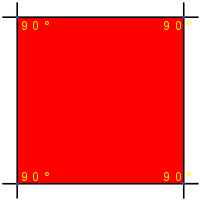Squares can tile the Euclidean plane with 4 around each vertex, with each square having an internal angle of 90°. The Schläfli symbol is {4,4}.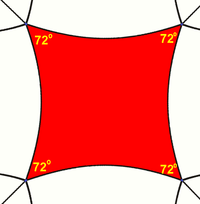Squares can tile the hyperbolic plane with 5 around each vertex, with each square having 72-degree internal angles. The Schläfli symbol is {4,5}. In fact, for any n ≥ 5 there is a hyperbolic tiling with n squares about each vertex.

## Crossed square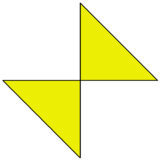Crossed-square

A crossed square is a faceting of the square, a self-intersecting polygon created by removing two opposite edges of a square and reconnecting by its two diagonals. It has half the symmetry of the square, Dih2, order 4. It has the same vertex arrangement as the square, and is vertex-transitive. It appears as two 45-45-90 triangle with a common vertex, but the geometric intersection is not considered a vertex.

A crossed square is sometimes likened to a bow tie or butterfly. the crossed rectangle is related, as a faceting of the rectangle, both special cases of crossed quadrilaterals.

The interior of a crossed square can have a polygon density of ±1 in each triangle, dependent upon the winding orientation as clockwise or counterclockwise.

A square and a crossed square have the following properties in common:

• Opposite sides are equal in length.
• The two diagonals are equal in length.
• It has two lines of reflectional symmetry and rotational symmetry of order 2 (through 180°).

It exists in the vertex figure of a uniform star polyhedra, the tetrahemihexahedron.

## Graphs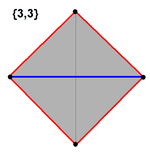The K4 complete graph is often drawn as a square with all 6 possible edges connected, hence appearing as a square with both diagonals drawn. This graph also represents an orthographic projection of the 4 vertices and 6 edges of the regular 3-simplex (tetrahedron).

1. Weisstein, Eric W. "Square." From MathWorld--A Wolfram Web Resource.
3. Chakerian, G.D. "A Distorted View of Geometry." Ch. 7 in Mathematical Plums (R. Honsberger, editor). Washington, DC: Mathematical Association of America, 1979: 147.
4. Josefsson, Martin, "Properties of equidiagonal quadrilaterals" Forum Geometricorum, 14 (2014), 129-144.
5. http://www2.mat.dtu.dk/people/V.L.Hansen/square.html
6. http://gogeometry.com/problem/p331_square_inscribed_circle.htm
7. Park, Poo-Sung. "Regular polytope distances", Forum Geometricorum 16, 2016, 227-232. http://forumgeom.fau.edu/FG2016volume16/FG201627.pdf
8. Zalman Usiskin and Jennifer Griffin, "The Classification of Quadrilaterals. A Study of Definition", Information Age Publishing, 2008, p. 59, ISBN 1-59311-695-0.
9. J. Wilson, Problem set 1.3, 2010
10. John H. Conway, Heidi Burgiel, Chaim Goodman-Strauss, (2008) The Symmetries of Things, ISBN 978-1-56881-220-5 (Chapter 20, Generalized Schaefli symbols, Types of symmetry of a polygon pp. 275-278)Wikimedia Commons has media related to Squares (geometry).

Fundamental convex regular and uniform polytopes in dimensions 2–10
Family An Bn I2(p) / Dn E6 / E7 / E8 / E9 / E10 / F4 / G2 Hn
Regular polygon Triangle Square p-gon Hexagon Pentagon
Uniform polyhedron Tetrahedron OctahedronCube Demicube DodecahedronIcosahedron
Uniform 4-polytope 5-cell 16-cellTesseract Demitesseract 24-cell 120-cell600-cell
Uniform 5-polytope 5-simplex 5-orthoplex5-cube 5-demicube
Uniform 6-polytope 6-simplex 6-orthoplex6-cube 6-demicube 122221
Uniform 7-polytope 7-simplex 7-orthoplex7-cube 7-demicube 132231321
Uniform 8-polytope 8-simplex 8-orthoplex8-cube 8-demicube 142241421
Uniform 9-polytope 9-simplex 9-orthoplex9-cube 9-demicube
Uniform 10-polytope 10-simplex 10-orthoplex10-cube 10-demicube
Uniform n-polytope n-simplex n-orthoplexn-cube n-demicube 1k22k1k21 n-pentagonal polytope
Topics: Polytope familiesRegular polytopeList of regular polytopes and compounds